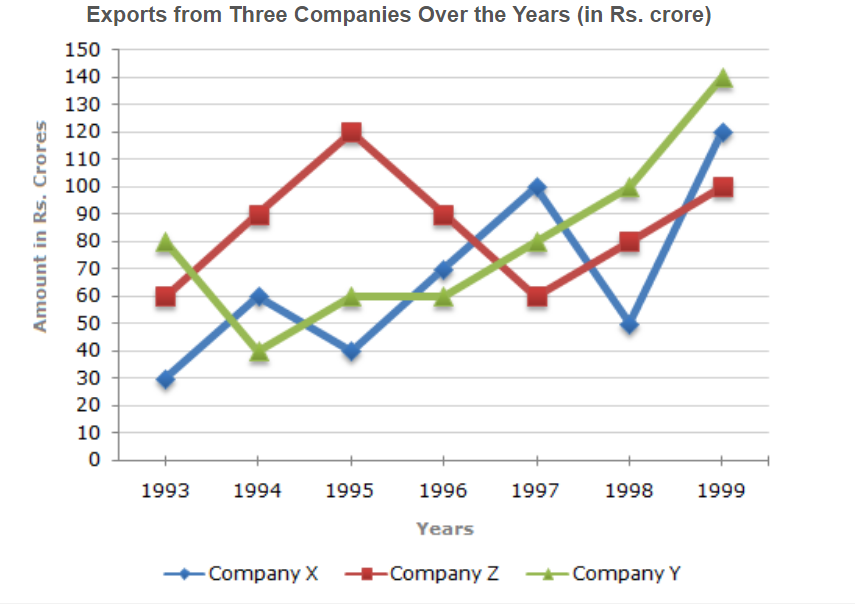# E-PolyLearning

 1. Study the following line graph and answer the question. For which of the following pairs of years the total exports from the three Companies together are equal?a. 1995 and 1998 b. 1996 and 1998 c. 1997 and 1998 d. 1995 and 1996

 2. Study the following line graph and answer the question. Average annual exports during the given period for Company Y is approximately what percent of the average annual exports for Company Z?a. 87.12% b. 89.64% c. 91.21% d. 93.33%
 3. Study the following line graph and answer the question. In which year was the difference between the exports from Companies X and Y the minimum?a. 1994 b. 1995 c. 1996 d. 1997
 4. Study the following line graph and answer the question. What was the difference between the average exports of the three Companies in 1993 and the average exports in 1998?a. Rs. 15.33 crores b. Rs. 18.67 crores c. Rs. 20 crores d. Rs. 22.17 crores
 5. Study the following line graph and answer the question. In how many of the given years, were the exports from Company Z more than the average annual exports over the given years?a. 2 b. 3 c. 4 d. 5